#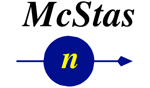In this document, we demonstrate how iFunc, iFit/Optimizers and iData objects can all be used transparently to simulate and optimize McStas/McXtrace neutron and X-ray ray-tracing Monte-Carlo instrument models.

To import a McStas simulation results, just use
`>> results = iData('single_detector_file')>> results = iData('directory')                 % import everything in the directory, including sim files (files may imported more than once)>> results = iData('scan_directory/mcstas.dat') % for scans>> results = iData('scan_directory/mcstas.sim') % for all files from the directory, uniquely.`
which returns a single or an array of iData object(s), that you can plot with e.g. subplot.
The resulting McStas data has an additional Parameters field which holds the instrument parameters used for the simulation.
`>> results(1).Parameters`

### Building an instrument model

The syntax for building a McCode (McStas for neutrons or McXtrace for X-rays) instrument model is:

`>> model = mccode('instrument');	% uses the given instrument file (*.instr)>> model = mccode('gui');		% lists all available instruments to select one>> model = mccode('');			% pop-up a file selector to select an *.instr file>> model = mccode('defaults');		% uses the templateDIFF instrument as example `
which compile the instrument, and create an iFunc model (in fact an iFunc_McCode flavour).
When the instrument is not found in the current directory, it is searched in the McStas/McXtrace library (may be specified with the MCSTAS and MCXTRACE environment variables), and eventually copied locally, which triggers its compilation.

In addition, it is possible to select an instrument from the McStas/McXtrace installation with:
`model = mccode('gui')`
You may even assemble these models as iFunc arrays to iteratively perform series of simulations, or use arithmetic operations to combine models (see the iFunc documentation).

The resulting 'model' object can be forced into an iFunc_McCode one (in case its flavour was lost) with:
• model = iFunc_McCode(model)
This type of sub-class (derived from iFunc), provides all iFunc methods, but also specific methods adapted to McCode simulations:
• plot(model)
plot the model geometry in 3D.
• feval(model)
evaluate the model using its current parameter values and settings. The returned data is an array for the selected or last Monitor value. Other monitors are available as iData objects in model.UserData.monitors
• edit(model)
edit the instrument source code. Do not forget to save the file before closing the editor. If changes are done, the object is updated, and the instrument is re-compiled.
• dialog(model)
displays a table where you can change the parameter/configuration values. Instrument parameters (scalars) can be given as a single scalar, or a vector (for scans). The calculation starts when you close the table. To Cancel the computation, select the contextual menu (right mouse button) CANCEL item, or press Ctrl-C at the prompt. The resulting monitors are displayed when the simulation end.
• publish(model)
create a full readable report as an HTML web page, including the instrument model view, results, and code.

It is also possible to finely tune the instrument configuration using standard McStas/McXtrace options with the syntax:
`>>  mccode('instrument', options)`
and the following options:
 options.dir directory where to store results, or set automatically (string) ; the last simulation files are stored therein 'sim'. options.ncount number of neutron events per iteration, e.g. 1e6 (double). options.mpi number of processors/cores to use with MPI on localhost or cluster (integer) ; when MPI is available, and mpi options is not given, all cores are then used. Use options.mpi=1 for a serial calculation. options.machines file name containing the list of machines/nodes to use (string), as often found in cluster job schedulers. options.seed random number seed to use for each iteration (double) options.gravitation 0 or 1 to set gravitation handling in neutron propagation (boolean) options.monitor a single monitor name pattern to read, or left empty for the last (string). The whole monitors are available as iData objects in the model.UserData.monitors field. Wildcard expression can be used. See below for more advanced customisation. options.mpirun set the executable path to 'mpirun', or automatically found when not set. Set it to 'none' or set options.mpi=1 to not compile with MPI. options.compile 0 or 1 to force re-compilation of the executable.

The options can be given as a structure or as a string, e.g. 'ncount=1e6; compile=1'.

NOTE: if the instrument can not be built, force the compilation with:
`>>  mccode('instrument', 'compile=1')`
as a previous executable may be in the way, and block the generation of the new model.

The scalar instrument parameters of type 'double' are used as model parameters.
Other parameters (e.g. of type string and int) are stored as a structure in:
• model.UserData.Parameters_Constant
The options ncount, seed, gravitation, monitor can be changed after the model creation in the UserData area of the object e.g.:
• model.UserData.options.gravitation=1;
• model.UserData.options.monitor='*Theta*';

#### Specifying which monitor to use

The default behaviour is to leave options.monitor left unset at the model creation, so that all monitors are read when simulating instruments. The last monitor is then used as model value. This provides maximum information, but the processing overhead time is increased due to more data handling compared to a single/restricted monitor name selection.
• model=mccode('templateDIFF');  % same as mccode('defaults')
In order to improve the computational efficiency, it is advised to specify which files have to be imported to define the model value by giving a file name or pattern (wildcard patterns can be used). This specification can be done either when building the model:
or afterwards:
• model=mccode('templateDIFF');
• model.UserData.options.monitor='*BananaPSD*'
which in both cases will select monitor files which match the given string as an exact file name or match the pattern when using a wildcard expression ( '*Theta*' ). In case the selection brings multiple files, the last one is used. When the monitor name selection does not match any file, or is given as empty, all monitors are imported, and the last one in the list is used.

All selected monitors are imported as iData object(s), in:
• model.UserData.monitors
In practice, you will often either not specify the 'monitor' at the model creation to leave the system select the last one, or indicate a specific monitor name/pattern of your choice.

Customize the model value
In addition, you may customize how to use the monitor data either by adding expression after the initial filename/pattern, or by extending the model after its creation.

The monitor to use as optimization criteria (options.monitor) can be part of a more complex expression, which still must start with the file name/pattern to select the monitor(s) and then refer to itself with the 'signal' variable (which are iData object(s)), such as in the following example where we sum all selected monitors from the pattern (when more than one are found), and define the criteria as Amplitude/width² (for a single peak):
`>> options.monitor='*Banana_Theta*; signal=plus(signal); signal=max(signal)/std(signal)^2;'>> model=mccode('templateDIFF', options)`
The options.monitor can be given in a compact way if the file pattern and the expression are enclosed in single or double quotes, such as in:
• model=mccode('templateDIFF','monitor="*Theta* signal=max(signal)/std(signal)^2"');
An other possibility (less recommended) is to extend the model after its creation:
• model = mccode('templateDIFF','monitor=Diff_BananaPSD*');
• model = model + 'signal=max(signal)/std(signal)^2';

#### Requirements

As iFit contains powerful import routines, that support McStas/McXtrace data files, it is straight forward to launch a McStas/McXtrace instrument simulation from Matlab and get back the simulation results. To do so, you will obviously need to have iFit installed (refer to the Install page), as well as McStas (refer to the McStas web site) or McXtrace. When used with the standalone version, no Matlab license is needed. To use all the CPU cores, you need to have installed an MPI library, e.g. OpenMPI.

### Running a single simulation

The model value is corresponds with the 'options.monitor' selected, i.e. the last one, or a specific name pattern. This selection can be changed any time without rebuilding the model (see above).

The instrument can be evaluated with given parameters and optionally axes:
`>> results = feval(model);			% return the monitor, using current/default parameters>> results = feval(model, parameters);		% return the monitor as an array using guessed axes>> results = feval(model, parameters, x,y,...);	% return the monitor as an array, using specified axes>> results = feval(model, parameters, nan);	% return the raw monitor as an array`
but it may be more convenient to manipulate the model value as iData objects:
`>> result = iData(model, parameters);		% return monitor interpolated onto guessed axes>> result = iData(model, parameters, nan);	% return raw monitor as an iData object>> result = iData(model, parameters, x,y,...);	% return monitor interpolated onto axes x,y,...>> plot(result);>> plot(model.UserData.monitors)`
where parameters is a structure, or string, or vector that holds parameter names and values, e.g.
• model=mccode('templateDIFF');
• parameters.RV=1;
• parameters.lambda=2.36;
• value = iData(model, parameters);
or in a more compact way, giving parameters as a single string with members assigned with '=' and separated with the ';' character. An empty parameter value (e.g. []) will compute the model evaluation using current/default parameters.

In the following example, we plot the model value (raw monitor), and then all the available monitors in model.UserData.monitors
• model= mccode('templateDIFF');
• value = iData(model, 'RV=1; lambda=2.36', nan)  % the last monitor
`value =  iData (methods,doc,plot,plot(log),values,more...) 2D object:    [Tag] [Dimension]                                     [Title] [Last command]          [Label/DisplayName]`
`  iD61442    [51 170]   'templateDIFF.instr McCode [... "signal"' iD61442=setalias(iD6... templateDI/templateDI...`
`model.UserData.monitors =  array [5  1] iData (methods,doc,plot,plot(log),values,more...) object:Index     [Tag] [Dimension]                                     [Title] [Last command]          [Label/DisplayName]    1   iD61449     [40 40] 'mccode.sim McCode sim file ... "Data ..."' iD61449=load(iData,'... Diff_Mono_XY    2   iD61457      [40 1] 'mccode.sim McCode sim file ... "Inten..."' iD61457=load(iData,'... Diff_Mono_Lambda    3   iD61459      [40 1] 'mccode.sim McCode sim file ... "Inten..."' iD61459=load(iData,'... Diff_Sample_Lambda    4   iD61461     [340 1] 'mccode.sim McCode sim file ... "Inten..."' iD61461=load(iData,'... Diff_BananaTheta    5   iD61463    [25 170] 'mccode.sim McCode sim file ... "Data ..."' iD61463=load(iData,'... Diff_BananaPSD`
You can equally set the non numerical scalar parameters (e.g. strings and integers):
model.UserData.Parameters_Constant.powder='Na2Ca3Al2F14.laz'
The unspecified instrument parameters are used with their default values (when defined).

The model value, and the raw monitors in model.UserData.monitors, in the form of iData objects have an additional Parameters field which holds the instrument parameters used for the simulation.
`>> value.Parameters>> get(model.UserData.monitors(1),'Parameters')`
Usual data analysis (display, mathematics, fit, export) can be performed (refer to the iFit and iData documentation).

### Getting instrument information and Displaying the instrument geometry

To display the instrument geometry, use the syntax: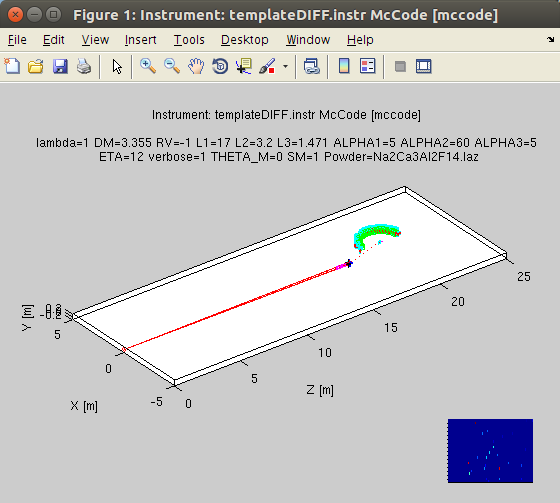`>> model = mccode('defaults')>> plot(model);`
which open a geometrical representation of the instrument. The current model parameters are used. It can be given as well a set of parameter values:
`>> plot(model, parameters);`
An empty parameter argument requests to use the current value. In addition, this routine returns the list of components, and can be given more options (3rd argument), as 'html','png', and 'x3d'.
`>> plot(model, [], 'html png x3d');`
to generate hardcopy files and geometry rendering. The generated filenames are then displayed.

In order to get information about the instrument, especially its input parameters, you can access the model.UserData area:
`>> model.UserDataans =         instrument_source: [1x7011 char]    instrument_executable: [1299344x1 double]           instrument_exe: 'templateDIFF.out'          instrument_info: [1x960 char]                  options: [1x1 struct]               Parameters: [1x1 struct]      Parameters_Constant: [1x1 struct]                 monitors: [5x1 iData]`
and especially the instrument_info and instrument_source, which you can view with e.g:
• disp(model.UserData.instrument_info)
or even in a separate window:
`>> TextEdit(model.UserData.instrument_source)>> TextEdit(model.UserData.instrument_info)`

### Running series of simulations (scans)

You may scan the model parameters when they are assigned as vector.
• model=mccode('templateDIFF');
• parameters.RV = 0.5:.05:1.5;
• scan = iData(model, parameters);
A more compact way can be used, using explicit scan values and axes:
• model=mccode('templateDIFF');
• scan = iData(model, 'RV=[0.5 1 1.5]', -.15:.05:.15, -5:50);
The argument returned is an iData object array containing the model monitor as a function of the scanned parameters. You may compute the integral value with the 'sum' method:
`>> integral = sum(scan, 0);`
which can be directly plotted with e.g.
`>> plot(integral);     % simple plot, or median surface for multidimensional scans>> scatter3(integral); % coloured points (possibly in 2D,3D)>> plot3(integral);    % coloured lines in 2D, or volume for multidimensional scans>> slice(integral);    % only for 3D scans, use slice-o-matic for volume inspection`
You may as well concatenate the scan steps into a higher dimensionality volume using e.g. the iData/cat method:
`>> catenated = cat(scan, 2);`
`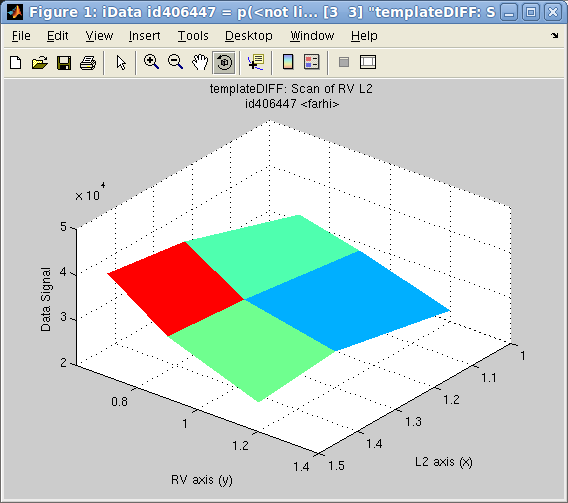`
Multidimensional scans are also possible. In the following example, we launch a quick 2D scan with parameters specified as a structure:
`>> model = mccode('templateDIFF','monitor=Diff_BananaTheta');>> scan = iData(model, struct('RV',[0.7 0.9 1.2],'L2',[1 1.3 1.5]), nan);`
Then we may plot, as a 2D surface, the integral value for the model value monitor:
`>> plot(iData(sum(scan,0)));`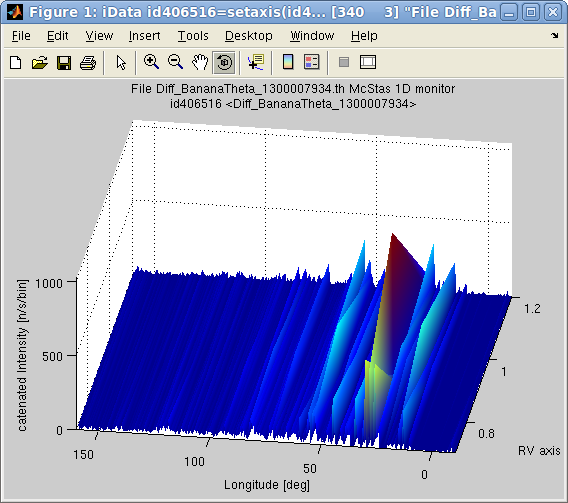You may look at the instrument parameters used for the scans, e.g.
`>> get(scan,'Parameters.RV')`
Warning: Beware of the number of iterations required to scan large dimensionality spaces. In practice you should avoid spaces above 2 or 3 scanned parameters. Exceeding this will undoubtedly require very long computation times, and large memory storage.

You may also scan the non numerical parameters with e.g. for the 'powder' string parameter:
• scan=[];
• for vector = {'Na2Ca3Al2F14.laz','Al.laz','Ge.laz'}; model.UserData.Parameters_Constant.powder = vector{1}; scan = [ scan iData(model, [], nan) ]; end
• subplot(scan)

#### Running series of simulations (single step and scans): graphical user interface

A user interface can be used to enter most McStas/McXtrace configuration and instrument parameters. To display it, use:
• dialog(model)
which shows a table where instrument parameters can be specified as scalars (e.g. 1), vectors (e.g. [1 2 3] or 0:0.5:5 or linspace(0,5,10) ) or strings ('Al.laz' for the additional non variable parameters such as file names, etc).

### Optimizing instrument parameters

The optimization of instrument parameters is performed as simply as a single simulation, using the fmax method to maximize the monitor value (you may similarly use fmin to minimize the model value).
`>> best_parameters = fmax(model);		% maximize with the current/default parameters>> best_parameters = fmax(model, parameters);	% maximize with given initial parameters`
The parameters, as a vector, string or named structure, are initiated to their starting value, from which the optimization proceeds. An empty value requests to use the current/default values. Only numerical parameters can be optimized. Also, it is highly encouraged to use bounded parameters (that is define constraints, see below), else the optimizer can get lost, produce un-meaningful results, or return NaN parameter values.

You can tune the optimizer configuration, as explained in the iFunc documentation, with syntax:
`>> best_parameters = fmax(model, parameters, options);`
as well as any other optimizer configuration fields such as
• options.TolFun ='1%'     % stop when criteria changes are smaller that 1%
• options.TolX ='0.1%';       % stop when parameter changes are smaller that 0.1%
• options.Display='final';
• options.OutputFcn='fminplot'; % plots the criteria and parameters evolution during the optimization.
• options.optimizer='fminpso'
• options.MaxFunEvals=100
The options can be given as a structure or assembled into a string. The default optimizer configuration is used when not specified (see the Optimizers page for more details). The optimizer routine is automatically guessed, but can be forced (see example below):
• fminpso: Particle Swarm Optimization
• fminpowell: Powell with Coggins line search
• fminhooke: Hooke-Jeeves direct search
• fminralg: Shor R-algorithm (with at least 2 parameters)
• fminsimpsa: Simplex/simulated annealing
• fminimfil: Unconstrained Implicit filtering
Eventhough shown as 'fminXXX' methods, the iFunc/fmax method in use here will make sure to maximize the monitor value by using the specified optimiser.

It is highly recommended to request the optimisation using the raw monitors. As indicated above, the axes are specified as additional arguments after the options:
`>> best_parameters = fmax(model, parameters, options, x, y, ...);`
and in particular you should probably use:
`>> best_parameters = fmax(model, parameters, options, nan);	% use raw monitors`
The optimization returns the best parameter guess, as well as the monitor value for this solution.

In the following example, a McStas/McXtrace model is assembled, specifying which monitor to use as model value. Then all parameters are fixed except RV and L2 which are bound (see section below). Last, the optimizer is explicitly indicated (else an automatic guess is made) as well as a request for a plot during the optimisation. In addition, similarly with other iFit optimization methods, the optimizer final status and optional returned information can be obtained with e.g.
`>> model = mccode('templateDIFF','monitor=*Theta*');	% monitor contains 'Theta'>> fix(model, 'all'); 				% fix all but RV L2>> model.RV='free'; model.RV=[0.5 0.8 1.5];	% set RV bounds and value in a single assignment>> model.L2='free'; model.L2=[1 3 4];>> [parameters, fval, status, output]=fmax(model,[], 'optimizer=fminimfil; OutputFcn=fminplot', nan);`
where output stores most of the optimization process data, including uncertainty on the best parameter values, output.parsHistoryUncertainty and output.parsHessianUncertainty.

To abort the optimization, just close the optimization plot window (or click the STOP button). The actual end occurs after a few more iterations.

You can then plot the monitor(s) for the optimised parameters with:
• model(parameters, nan);  % evaluate the best solution
• figure; subplot(model.UserData.monitors)
Warning: as McStas/McXtrace use a Monte-Carlo technique (which means 'random'), the criteria is a noisy function. The stop conditions must then usually be quite relaxed for the optimization to end, or the number of ncounts must be large enough to minimize the statistical uncertainty. TolFun='1%' and TolX='0.1%' are fair choices. Also, we recommend to constrain parameter ranges (see below). Also, increasing the 'ncount' (which is 1e5 by default) may help the optimizer to search in a noisy criteria landscape.

### Optimizing instrument parameters with constraints

It is quite usual the restrict the parameter search to specified ranges, and fix some of the parameters. As the model is an iFunc object, you may fix some parameter with any of:
• mlock(model, 'par')
• model.par='fix'
or alternatively clear (free) them with:
• munlock(model, 'par')
• model.par='free'
You may as well bound a parameter with: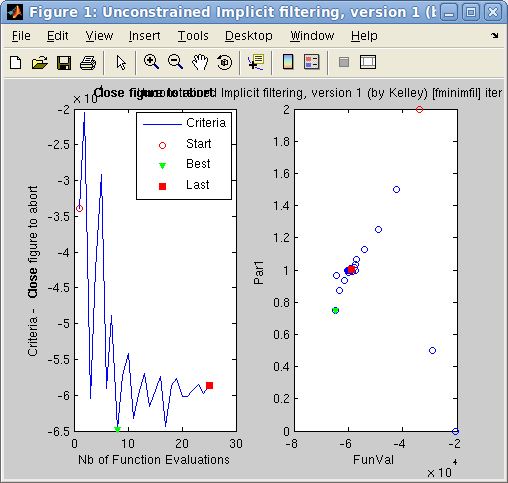• xlim(model, 'par', [min max])
• model.par = [ min max]
or even bound a parameter and set its value in a single line:
• model.par = [ min value max]
In the following example we optimise the monochromator radius of curvature in the templateDIF diffractometer, in order to get the maximum flux in the diffractogram:
`>> model = mccode('templateDIFF','monitor=*Theta*');	% monitor contains 'Theta'>> fix(model, 'all'); 				% fix all>> model.RV='free'; model.RV=[0.5 1 1.5];	% set RV free, bounds and value in a single assignment>> [parameters, fval, status, output]=fmax(model,[], ...  'optimizer=fminpso; OutputFcn=fminplot;TolFun =5%;TolX=5%;ncount=1e5;MaxFunEvals=100', nan);`
You can then plot the monitor(s) for the optimised parameters with:
• model.RV
`	ans=           0.8621`
• model(parameters, nan);  % evaluate the best solution
• figure; subplot(model.UserData.monitors)
We strongly recommend to limit the parameter search within boundaries during optimizations.

### Preparing data files for McStas components

#### PowderN component: elastic coherent scattering from a powder

The PowderN component can use both LAU ('Laue' files from Crystallographica for 'Single_crystal') and LAZ ('Lazy/Pulvrx' from e.g. ICSD for PowderN). These files can be prepared using 'cif2hkl'. That tool is also included with the McStas distribution as executable.

The cif2hkl syntax is:
• [file_out, result] = cif2hkl(file_in, file_out, lambda, mode, verbose)
The iFit version can use as first argument 'file_in':
• a CIF file (does not support mmCIF)
• a CFL file (FullProf)
• a INS/SHX/RES file (ShelX)
• a chemical formula with syntax e.g. 'cod: La Mn O3' (Hill notation)
• a Crystallography.net ID with syntax e.g. 'cod: 1532356'

The file_out argument specifies the output file name to use. If left empty, it adds an extension to the initial file.

The lambda argument specifies the wavelength to use for the definition of the HKL cut-off. Default is 0.5 (in Angs).

The 'mode' argument can be given as
• 'p' for powder mode to generate a LAZ file
• 'x' for single crystal mode to generate a LAU file
For example:
• cif2hkl('Al.cif')
• cif2hkl('Al.cif', [], [], 'x')
WARNING: it is essential to check the output file (text), especially the density, molar weight, cross sections, etc.

#### Single_crystal component: elastic coherent scattering from a single crystal

The procedure to generate LAU files for the Single_crystal component is similar as the one above for PowderN. Just use 'x' as mode argument.

WARNING: it is essential to check the output file (text), especially the density, molar weight, cross sections, etc.

#### Isotropic_Sqw component: coherent and incoherent scattering from an isotropic density material (liquid, gas, powder, amorphous)

The Isotropic_Sqw component is able to simulate all neutron scattering contributions for isotropic density materials: elastic, inelastic, coherent, incoherent. The main idea is to first generate a 2D iData object, then cast it as a iData_Sqw2D flavour and export it in the 'mcstas' format.
• d = iData('file');
• d = iData_Sqw2D(d);
• saveas(d, '', 'mcstas');
The initial 2D data set may for instance be obtained from:
• a measured S(q,w) (after reduction and correction)
• a molecular dynamics treated with e.g. MDANSE to obtain the coherent and incoherent S(q,w)
• a powder average of a 4D model [iFunc_Sqw4D and powder method]
WARNING: it is essential to check the output file (text), especially the density, molar weight, cross sections, etc.

Let's review in details the different strategies to generate these files:

coherent scattering from 4D S(q,w) models

The Sqw4D models, e.g. phonons [from DFT, analytical, cubic, ...], spin-waves [SpinW, SpinWave] can be evaluated on a whole Brillouin zone and averaged along momentum transfer to generate a 'powder' representation of the crystal. We also recommend that you have a look at the Neutron Scattering page for more details about the theory.

We first create a 4D S(q,w) model, such as:

 Command to generate a S(hklw) 4D model Description s = sqw_sine3d(20); a simple acoustic branch ranging up to 20 meV s = sqw_sine3d([ 15 20 ]); a simple optic branch oscillating from 15 to 20 meV s = sqw_spinw( sw_model('squareAF',2,0) ); a SpinW model, here AF. Any other 'sw' model can be used. s = sqw_spinwave('MnFe4Si3.txt'); a SPINWAVE (LLB) model s = sqw_vaks('KTaO3'); a Vaks perovskite, with KTaO3 parameters s = sqw_cubic_monoatomic([ 3 3 ]); a simple cubic monoatomic, with C1/C2=3 E0=3 s = sqw_phonons('Al.cif','metal'); a DFT phonon (lattice dynamics). Can also import e.g. PhonoPy calculation.

Then convert the model to a powder one (2D Sqw):
• p = powder(s);
Finally, evaluate the model on given momentum and energy axes, and export:
• q = 0:0.05:10; % Angs-1
• w = 0:0.25:50; % meV
• d = iData(p, [], q, w); % with default model parameters
• saveas(d, 'myPowder.sqw','sqw');
incoherent scattering from lattice dynamics

In the case of lattice dynamics, the vibrational density of states can be computed directly:
• s = sqw_phonons('Al.cif','metal','emt');
• g = dos(s);
The so-called 'multi-phonon' expansion [Sjolander 1958] allows to estimate the inelastic incoherent scattering contribution from the density of states, possibly specifying the mass and temperature (when not found in the model). The Debye-Waller coefficient can also be specified.
• inc = incoherent(g, 'T', 10, 'DW', 0.05); % all multi-phonon terms, to be summed-up
• g = plus(inc);
• saveas(g, 'Al_pow_inc_10.sqw','sqw');
coherent and incoherent scattering from molecular dynamics

If you have access to a molecular dynamics trajectory, we recommend that you use MDANSE. The procedure is as follows:
1. First convert the input trajectory into a NetCDF file (MDANSE/File/Trajectory converters menu).
2. Then open that file.
3. Double click the new entry in the Data panel (lower left). A list of applicable items shows in the upper Plugins panel.
4. Open the Analysis item, then the Scattering one.
5. Double click the Dynamic Coherent Structure Factor (DCSF).
6. In the 'q vectors' panel, select 'New' and use the 'spherical_lattice' generator up to e.g. shells 100 (in nm-1, i.e. 10 Angs-1). Give it a name, Generate and Save.
7. Back in the DCSF Algorithm dialog, set the output filename (e.g. DCSF), and click Run. A NetCDF file is created.
8. Perform exactly the same steps for the Dynamic Incoherent Structure Factor (DISF).
9. Close MDANSE.
Then import the coherent and incoherent NetCDF files from MDANSE, then save it for McStas, e.g.:
• d = iData_Sqw2D('DCSF.nc')
• saveas(d, 'DCSF.sqw','sqw')
incoherent scattering from experiment/density of states

If you perform a neutron scattering experiment on a spectrometer, you should reduce and correct the measurement data file. Then convert it to S(q,w) and/or estimate the vibrational density of states. Finally, follow the same procedure as the one for lattice dynamics.

The so-called 'multi-phonon' expansion [Sjolander 1958] allows to estimate the inelastic incoherent scattering contribution from the density of states, possibly specifying the mass and temperature (when not found in the model). The Debye-Waller coefficient can also be specified. We assume the vDOS has been imported as variable 'g':
• g = iData_vDOS('dos_file');
• inc = incoherent(g, 'T', 10, 'DW', 0.05); % all multi-phonon terms, to be summed-up
• g = plus(inc);
• saveas(g, 'inc_10.sqw','sqw');
coherent scattering from experiment

If you perform a neutron scattering experiment on a spectrometer, you should reduce and correct the measurement data file. Remove the empty cell/container contribution. Then convert it to S(q,w), possibly applying a radial momentum average. Estimate its density of states, and the incoherent scattering (see above). Subtract the incoherent scattering from the total scattering, to get an estimate of the coherent scattering alone. Make it an iData_Sqw2D object, and save it as a 'sqw' format file.

total scattering from experiment

If you perform a neutron scattering experiment on a spectrometer, you should reduce and correct the measurement data file. Remove the empty cell/container contribution. Then convert it to S(q,w), possibly applying a radial momentum average. When using the Isotropic_Sqw, set the sigma_inc component parameter to 0, and the sigma_coh as the total scattering cross-section.

#### Single_crystal_inelastic component: coherent and incoherent scattering from a single crystal

The Single_crystal_inelastic component is an experimental contribution which allows to estimate all neutron scattering contributions for single crystal materials: elastic, inelastic, coherent, incoherent.

The main idea is to generate a 4D model [iFunc_Sqw4D], evaluate it to produce a iData_Sqw4D which is then exported in the 'mcstas' format.
Refer to the Models page to get an overview of all available 4D S(q,w) models.
• s = sqw_spinw('defaults')
• d = iData(s); % use default grid for axes
• saveas(d, 'sx_coh.sqw4', 'mcstas');

E. Farhi - iFit/McStas - Nov. 27, 2018 2.0.2 - back to Main iFit Page